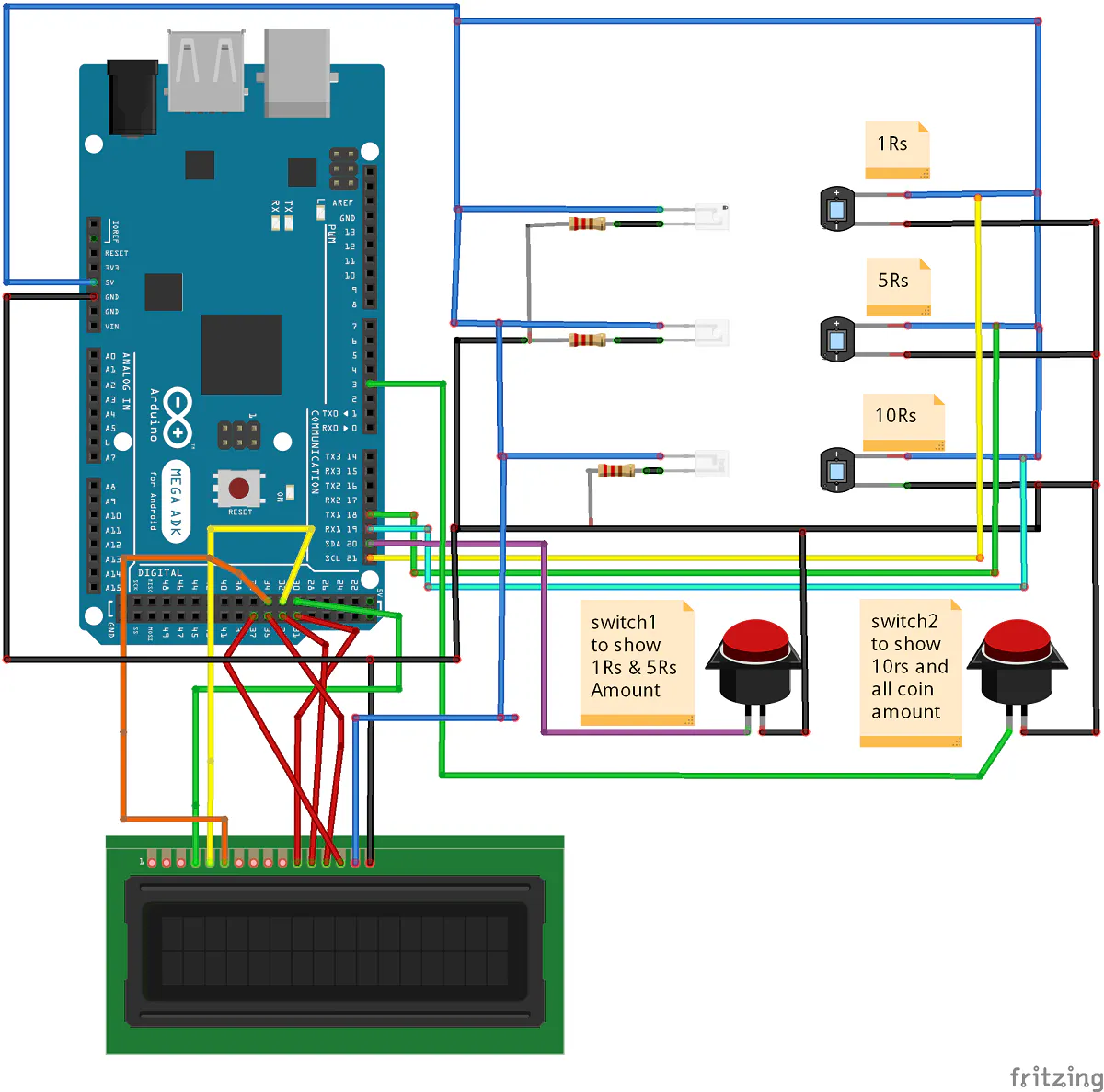Project showcaseCoin Detector and Amount Counter © CC BY

It counts the amounts of 1Rs, 5Rs and 10Rs coins as well as the total amount of coins.

• 13,446 views
• 10 respects

Apps and online services

Coin counter and amount counter is the project in this project number of coin inserted are first sorted through the slider and holes the there are IR led sensors to sense the coin then an interrupt signal is send to Arduino of that sense coin and further calculation are done and displayed on lcd 16x2 and serial monitor.

Code

coin detector counter and amount counterC#
/*
COIN DETECTOR amount counter USING INTERRUPT
DETEECT THE COIN USING INFRARED LED
INTERRUPT PINS ARE CONNECTED TO THE CATHODE OF IR Recivers
USING ARDUINO MEGA 2560
*/

#include <LiquidCrystal.h>

LiquidCrystal lcd(30, 32, 34, 31, 33, 35, 37);
const byte interruptPin1 = 21;
const byte interruptPin2 = 18;
const byte interruptPin3 = 19;
const byte interruptPin4 = 20;
const byte interruptPin5 = 3;
volatile int value10 = 0;
volatile int value5 = 0;
volatile int value1 = 0;
volatile int valueswitch = 0;
volatile int countOne = 0 ;
volatile int countFive = 0;
volatile int countTen = 0;
volatile int TOTAL=0;
void setup() {
pinMode(interruptPin1, INPUT_PULLUP);
pinMode(interruptPin2, INPUT_PULLUP);
pinMode(interruptPin3, INPUT_PULLUP);
pinMode(interruptPin4, INPUT_PULLUP);
pinMode(interruptPin5, INPUT_PULLUP);
attachInterrupt(digitalPinToInterrupt(interruptPin1), coinOne , RISING);
attachInterrupt(digitalPinToInterrupt(interruptPin2), coinFive , RISING);
attachInterrupt(digitalPinToInterrupt(interruptPin3), coinTen , RISING);
attachInterrupt(digitalPinToInterrupt(interruptPin4), show_1_5, RISING);
attachInterrupt(digitalPinToInterrupt(interruptPin5), show_10, RISING);
lcd.begin(16, 2);

lcd.print("COIN DETECTOR &");
lcd.setCursor(1, 1);
lcd.print("AMOUNT COUNT");
delay(1200);
delay(1200);
delay(1200);

lcd.begin(16, 2);
lcd.print("INSERT THE COIN");

Serial.begin(9600);
Serial.println(" ");
Serial.print("COIN DETECTOR , SORT & AMOUNT COUNTER ");
}

void loop() {

delay(2000);
lcd.begin(16, 2);
lcd.print("INSERT THE COIN");

}

/*

INTERRUPT SERVICE ROUTINE

*/

void coinTen() {
countTen++;
value10 = (countTen * 10);
Serial.println("\n");
Serial.println(" 10Rs coin = \r ");
Serial.print(value10);
Serial.print("Rs");
Serial.println("\n");

}
void coinFive() {
countFive++;
value5 = (countFive * 5);
Serial.println("\n");
Serial.println(" 5Rs coin = \r ");
Serial.print(value5);
Serial.print("Rs");

}

void coinOne() {
countOne++;
value1 = (countOne);
Serial.println("\n");
Serial.println(" 1Rs coin = \r ");
Serial.print(value1);
Serial.print("Rs");
}
void show_1_5()
{
Serial.println("\n");
Serial.println("Number of 1Rs coins =  \r ");
Serial.print(countOne);
Serial.println("\n");
Serial.println("TOTAL 1Rs COINS =  \r ");
Serial.print(value1);
Serial.print("Rs");
Serial.println("\n");
Serial.println("Number of 5Rs coins =  \r ");
Serial.print(countFive);
Serial.println("\n");
Serial.println("TOTAL Rs COINS =  \r ");
Serial.print(value5);
Serial.print("Rs");
//coin of one Rs
lcd.begin(16, 2);
lcd.print("1Rs coin= ");
lcd.setCursor(11, 0);
lcd.print(value1);
// coin of five Rs
lcd.setCursor(0, 1);
lcd.print("5Rs coin =");
lcd.setCursor(11, 1);
lcd.print(value5);

}
void show_10()
{
Serial.println("\n");
Serial.println("Number of 10Rs coins =  \r ");
Serial.print(countTen);
Serial.println("\n");
Serial.println("TOTAL 10Rs COINS =  \r ");
Serial.print(value10);
Serial.print("Rs");
//coin of 10Rs
lcd.begin(16, 2);
lcd.print("1Rs coin= ");
lcd.setCursor(11, 0);
lcd.print(value10);
//TOTAL AMOUNT
TOTAL=(value1+value5+value10);
lcd.setCursor(0, 1);
lcd.print("TOTAL =");
lcd.setCursor(11, 1);
lcd.print(TOTAL);
Serial.println("\n");
Serial.println("TOTAL AMOUNT =  \r ");
Serial.print(TOTAL);
Serial.print("Rs");
}

SchematicsOctober 28, 2017

Members who respect this project

See similar projects
you might like

Arduino EMF Detector A.K.A Ghost Detector

Project showcase by Patel Darshil

• 4,957 views
• 14 respects

HomeLife Nightstand

Project showcase by Florian Siess

• 966 views
• 1 comment
• 6 respects

Smart 3D Printer Filament Counter (FilamentBot)

Project tutorial by ArtSuzhou

• 8,683 views
• 24 respects

Digital 3D Printer Filament Counter Using PS/2 Mouse

Project in progress by ArtSuzhou

• 8,441 views
• 32 respects

The Vibrating Pressure Temperature Altitude Tilt Detector

Project tutorial by Kutluhan Aktar

• 1,661 views